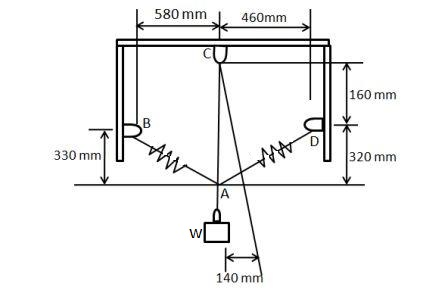# A block of weight ''W'' is suspended from a 500 ''mm'' long cord and two springs of which the...

## Question:

A block of weight W is suspended from a 500 mm long cord and two springs of which the unstretched lengths are 450 mm. Knowing that the constants of the spring are {eq}k_{AB} {/eq}= 1500 N/m and {eq}k_{AD} {/eq} = 500N/m, determine (a) the tension in the cord, (b) the weight of the block.## Spring constant:

The value of force required to deform the spring by unity within the spring is known as spring constant. The standard unit of spring constant is newton meter inverse. Spring constant of weak spring is less, and strong spring has high spring constant.

Become a Study.com member to unlock this answer! Create your account

Given data

• Unscratched length of the two springs, {eq}{L_s} = 450\;{\rm{mm}} {/eq}
• Spring constant of spring AB, {eq}{K_{AB}} =...Practice Applying Spring Constant Formulas

from

Chapter 17 / Lesson 11
3.3K

In this lesson, you'll have the chance to practice using the spring constant formula. The lesson includes four problems of medium difficulty involving a variety of real-life applications.